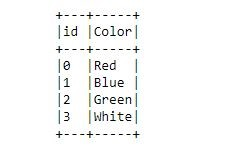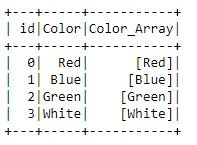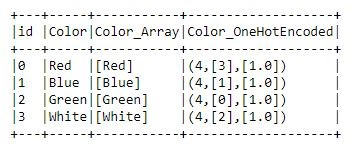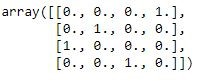PySpark One Hot Encoding with CountVectorizer

One Hot Encoding is an important technique for converting categorical attributes into a numeric vector that machine learning models can understand. In this article you will learn how to implement one-hot encoding in PySpark.

Getting Started

Before we begin, we need to instantiate a Spark SQLContext and import required python modules.

#Import PySpark libraries
import pyspark
from pyspark import SparkContext, SparkConf
from pyspark.sql import SQLContext

Next, we need to connect to a spark cluster (or your local spark) and instantiate a new SQLContext class since we will be working with spark dataframes.

#Connect to Spark
conf = SparkConf().setAppName("Vectorizer")
sc = SparkContext(conf=conf)

#Create an SQLContext class
sqlContext = SQLContext(sc)

Print the version of spark you are utilizing. This is important to know as functionality varies across spark versions. This article is written using spark version 2.3.2.

print("Spark Version: " + sc.version)
#Spark Version: 2.3.2

Create Spark DataFrame

In the next code block, generate a sample spark dataframe containing 2 columns, an ID and a Color column. The task at hand is to one hot encode the Color column of our dataframe. We call our dataframe, df.

df = sqlContext.createDataFrame([
(0, "Red"),
(1, "Blue"),
(2, "Green"),
(3, "White")
], ["id", "Color"])

Display the spark dataframe we have generated.

df.show(truncate=False)Convert String To Array

To run one-hot encoding in PySpark we will be utilizing the CountVectorizer class from the PySpark.ML package. One of the requirements in order to run one hot encoding is for the input column to be an array.

Our Color column is currently a string, not an array. Convert the values of the “Color” column into an array by utilizing the split function of pyspark. Run the following code block to generate a new “Color_Array” column.

from pyspark.sql.functions import col, split
df = df.withColumn("Color_Array", split(col("Color")," "))

df.show()Our data is now ready for us to run one-hot encoding utilizing the functions from the pyspark.ml package.

PySpark CountVectorizer

Pyspark.ml package provides a module called CountVectorizer which makes one hot encoding quick and easy.

Yes, there is a module called OneHotEncoderEstimator which will be better suited for this. Bear with me, as this will challenge us and improve our knowledge about PySpark functionality.

The CountVectorizer class and its corresponding CountVectorizerModel help convert a collection of text into a vector of counts. The result when converting our categorical variable into a vector of counts is our one-hot encoded vector. The size of the vector will be equal to the distinct number of categories we have. Let’s begin one-hot encoding.

Import the CountVectorizer class from pyspark.ml

#Import Spark CountVectorizer
from pyspark.ml.feature import CountVectorizer

Now, create a CountVectorizer class which we will call colorVectorizer. Some important parameters that we need to provide are the following:

• inputCol:  specifies the column to be one-hot encoded
• outputCol: the created column, this will be our one-hot encoded column.
• VocabSize: specifies how many words to keep in our vocabulary
• MinDF: specifies in how many rows does a word need to appear for it to be counted.
# Initialize a CountVectorizer.
colorVectorizer = CountVectorizer(inputCol="Color_Array", outputCol="Color_OneHotEncoded", vocabSize=4, minDF=1.0)

Next, call the fit method of the CountVectorizer class to run the algorithm on our text. The resulting CountVectorizer Model class will then be applied to our dataframe to generate the one-hot encoded vectors.

#Get a VectorizerModel
colorVectorizer_model = colorVectorizer.fit(df)

With our CountVectorizer in place, we can now apply the transform function to our dataframe. This function will use the Color_Array column defined as the input and output the Color_OneHotEncoded column.

df_ohe = colorVectorizer_model.transform(df)
df_ohe.show(truncate=False)We are done. The new added column into our spark dataframe contains the one-hot encoded vector. As we are using the CountVectorizer class and applying it to a categorical text with no spaces and each row containing only 1 word, the resulting vector has all zeros and one 1.

How do we extract the array into a numpy array for example? Do the following.

import numpy as np
x_3d = np.array(df_ohe.select('Color_OneHotEncoded').collect())
x_3d.shape
#(4, 1, 4)

Only run collect in pyspark if your master driver has enough memory to handle combining the data from all your workers. Otherwise, you would need to run a batch type method instead.

We obtained the Color_OneHotEncoded column into a 3d Array. We need to convert this into a 2D array of size Rows, VocabularySize.

Get the shape from our x_3d variable and obtain the Rows and VocabSize as you can see below. Then, reshape your array into a 2D array which each line contains the one-hot encoded value for the color input.

rows, idx, vocabsize = x_3d.shape
X = x_3d.reshape(rows, features)
X.shape
#(4, 4)Reverse One-Hot Encoding

Ok, we are done going from text to a one-hot vector. What if we needed to go the other way around? From a one-hot encoded vector to a text, Color in this case. For this, we need to build a reverse dictionary.

First, get the Colors from our dataframe by running a similar command as we did to get the One Hot vector array. The result is an array, therefore we run a list comprehension method to get a list of Colors.

Colors = np.array(df_ohe.select('Color').collect())
Colors = [str(c).strip() for c in Colors]
print(Colors)
#['Red', 'Blue', 'Green', 'White']

These correspond to each row of our X array. For each row, let's find the index of the array which has the One-Hot vector and lastly loop through their pairs to generate or index and reverse_index dictionary.

Use the where function in Numpy to get the location of the one-hot index.

np.where(X == 1)
#array([3, 1, 0, 2], dtype=int64)

Generate the word2int and reverse_word2int dictionaries.

reverse_word2int = {}
word2int= {}
for color, index in zip(Colors,list(np.where(X == 1))):
reverse_word2int[index] = color
word2int[color] = index

Let’s see if our dictionaries make sense. Let’s test the color Red getting its index in the one-hot vector and it’s reverse.

print(word2int['Red'])
print(reverse_word2int)
#3
#Red

To go from a one-hot encoding vector back to the label, all you need is the location of the one-hot vector (value of 1) within the array which is easy to obtain using Numpy. Then, utilize your reverse_word2int dictionary to obtain the label.

Conclusion

Spark is a powerful data processing engine and its ML library provides much needed functions to build machine learning models. In this article we saw how to implement one-hot encoding and reverse one-hot encoding using the CountVectorizer module. In other articles I will show how to use the OneHotEncoderEstimator module.